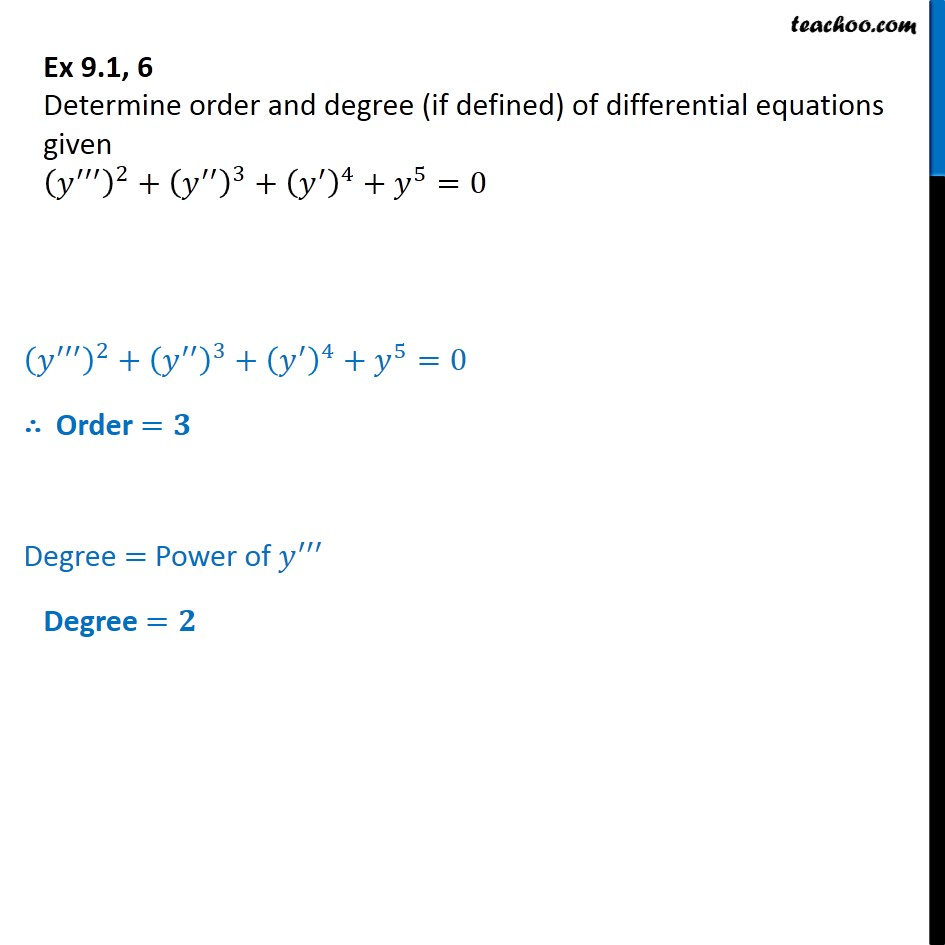Ex 9.1

Chapter 9 Class 12 Differential Equations
Serial order wiseLearn in your speed, with individual attention - Teachoo Maths 1-on-1 Class

### Transcript

Ex 9.1, 6 Determine order and degree (if defined) of differential equations given (y"')2 + (y")3 + (y')4 + y5 = 0 (y"')2 + (y")3 + (y')4 + y5 = 0 Order = Degree = Power of y" Degree =